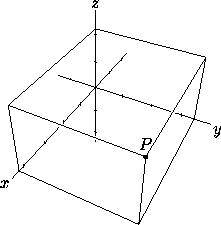# 9.1 Cartesian Coordinates in Space

In cartesian coordinates (or rectangular coordinates), a point P is referred to by three real numbers, indicating the positions of the perpendicular projections from the point to three fixed, perpendicular, graduated lines, called the axes. If the coordinates are denoted x, y, z, in that order, the axes are called the x-axis, etc., and we write P=(x,y,z). Often the x-axis is imagined to be horizontal and pointing roughly toward the viewer (out of the page), the y-axis also horizontal and pointing more or less to the right, and the z-axis vertical, pointing up. The system is called right-handed if it can be rotated so the three axes are in this position. Figure 1 shows a right-handed system. The point x=0, y=0, z=0 is the origin, where the three axes intersect.Figure 1: In cartesian coordinates, P=(4.2,3.4,2.2).

Next: 9.2 Cylindrical Coordinates in Space
Up: 9 Coordinate Systems in Space
Previous: 9 Coordinate Systems in SpaceThe Geometry Center Home Page

Silvio Levy
Wed Oct 4 16:41:25 PDT 1995

This document is excerpted from the 30th Edition of the CRC Standard Mathematical Tables and Formulas (CRC Press). Unauthorized duplication is forbidden.